1800-1023-196

+91-120-4616500

# Analysis of Cubic System

## Analysis of Cubic System

(1) Number of atoms in per unit cell

The total number of atoms contained in the unit cell for a simple cubic called the unit cell content.

The simplest relation can determine for it is,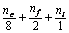Where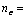Number of atoms at the corners of the cube=8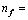Number of atoms at six faces of the cube = 6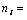Number of atoms inside the cube = 1

#### Cubic unit cell

nc nf ni Total atom in per unit cell
Simple cubic (sc) 8 0 0 1
body centered cubic (bcc) 8 0 1 2
Face centered cubic (fcc) 8 6 0 4

(2) Co-ordination number (C.N.): It is defined as the number of nearest neighbours or touching particles with other particle present in a crystal is called its co-ordination number. It depends upon structure of the crystal.

For simple cubic system C.N. = 6.

For body centred cubic system C.N. = 8

For face centred cubic system C.N. = 12.

(3) Density of the unit cell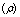: It is defined as the ratio of mass per unit cell to the total volume of unit cell.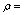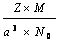Where Z = Number of particles per unit cell

M = Atomic mass or molecular mass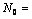Avogadro number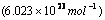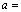Edge length of the unit cell=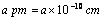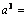volume of the unit cell

i.e.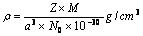The density of the substance is same as the density of the unit cell.

Packing fraction (P.F.): It is defined as ratio of the volume of the unit cell that is occupied by spheres of the unit cell to the total volume of the unit cell.

Let radius of the atom in the packing = r

Edge length of the cube = a

Volume of the cube V =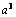Volume of the atom (spherical)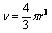Packing density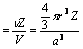###### Structure
r related to a Volume of the atom (n) Packing density % of void
Simple cubic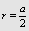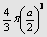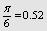100–52 = 48%
Face-centred cubic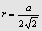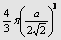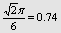100 – 74 = 26%
Body-centred cubic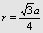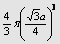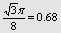100 – 68 = 32%

## NEET & AIIMS Exam Sample Papers

 AIIMS SAMPLE PAPERS View More NEET SAMPLE PAPERS View More